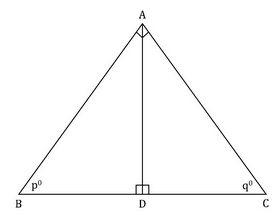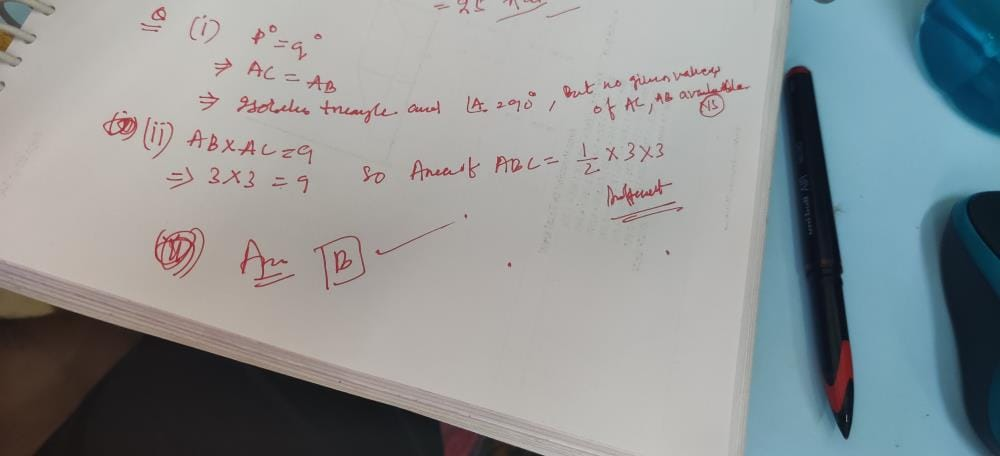From the given diagram, find the area of tria...

### Related TestFrom the given diagram, find the area of triangle ABC.
1. po = qo
2. The product of AB and AC is 9.
• a)
Statement (1) ALONE is sufficient, but statement (2) alone is not sufficient to answer the question asked.
• b)
Statement (2) ALONE is sufficient, but statement (1) alone is not sufficient to answer the question asked.
• c)
BOTH statements (1) and (2) TOGETHER are sufficient to answer the question asked, but NEITHER statement ALONE is sufficient to answer the question asked.
• d)
• e)
Statements (1) and (2) TOGETHER are NOT sufficient to answer the question asked, and additional data specific to the problem are needed.Preitee Ranjan PradhanView courses related to this question Explore GMAT courses
 Explore GMAT coursesView courses related to this question1 Crore+ students have signed up on EduRev. Have you?

• ### In triangle ABC (not shown), is the range of the angles of the triangle gre... more(Scan QR code)